Expression

If A=2 B=3 evaluate expression A(B+A) and multiply it by A

Result

x =  20

Solution:Leave us a comment of example and its solution (i.e. if it is still somewhat unclear...):Be the first to comment!To solve this example are needed these knowledge from mathematics:

Do you have a linear equation or system of equations and looking for its solution? Or do you have quadratic equation?

Next similar examples:

1. I thinkI think a number. When I multiply it by five, and after that I subtract 477, I get the same number as if I multiplied it twice. What number do I think?
2. Chestnuts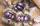Neil has 5-times chestnuts less than Adrian. Together they have 894 chestnuts. How many chestnuts has Neil and Adrian?
3. Textbooks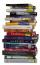After check of textbooks found that every 10-th textbook should be withdrawn. Together 58 textbooks were withdrawn. How many textbooks were in stock before withdrawn and how many after withdrawn?
4. What isWhat is the value of the smaller of a pair of numbers for which their sum is 78 and their division quotients are 0.3?
5. ProductResult of the product of the numbers 1, 2, 3, 1, 2, 0 is:
6. Written numberPlace+values x ten thousands =30 thousands
7. Computer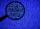A line of print on a computer contains 64 characters (letters, spacers or other chars). Find how many characters there are in 7 lines.
8. Icecreams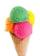Karthik bought 30 icecreams for his birthday .2/5 of them are chocolate icecreams. Find the number of chocolate icecreams he bought?
9. SummandOne of the summands is 145. The second is 10 more. Determine the sum of the summands.
10. What is missing (1000)What number is to add to get 1000?
11. Number unknownAdela thought the two-digit number, she added it to its ten times and got 407. What number does she think?
12. Seven timesWhich number seven times is just as higher as 27, how much is smaller than 29?
13. Unknown number xyzFind the number that its triple is 24. Solve by equation.
14. Eq1Solve equation: 4(a-3)=3(2a-5)
15. Hotel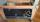The rooms in the mountain hotel are double and triple. Double rooms are 25 and triple are 17 more. How many rooms are there in this hotel?
16. If-then equationIf 5x - 17 = -x + 7, then x =
17. Roman numeralsWrite numbers written in Roman numerals as decimal.